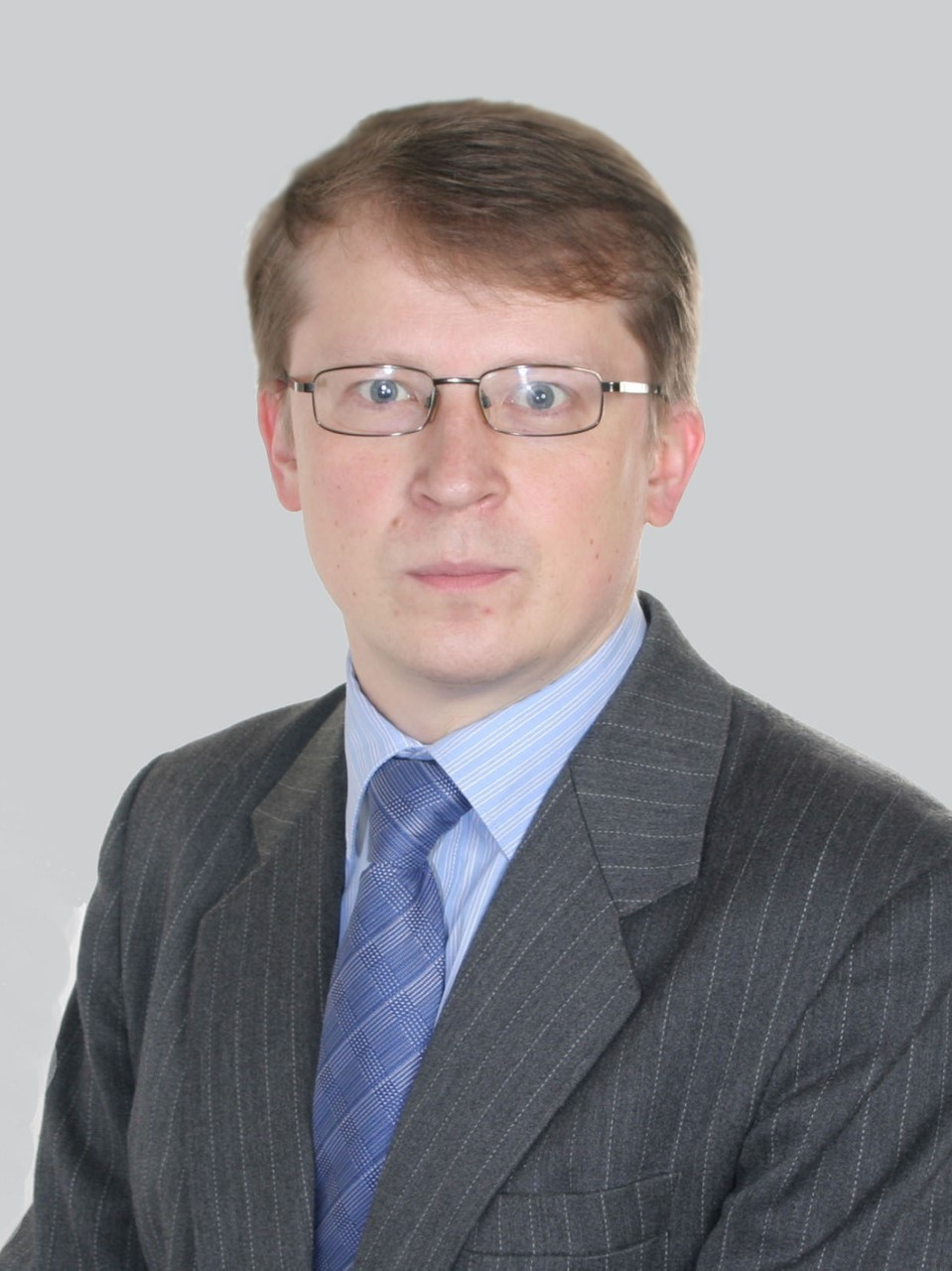Noncommutative elliptic theory
Noncommutative elliptic theory

Year 2018

Area of science:

Mathematics

Scientific direction:

Partial differential equations

The main goal of the project is to create a noncommutative elliptic theory for a new class of operators associated with the representation of a group by quantized canonical transformations on different varieties. We study the Fredholm property of the studied operators and index formulas are presented for them.

Key publications on the project:

• A. Y. Savin, E. Schrohe, B. Y. Sternin Elliptic operators associated with groups of quantized canonical transformations. Successes of Mathematical Sciences. Vol. 73, 2018.
• A. Savin, E. Schrohe, B. Sternin Elliptic operators associated with groups of quantized canonical transformations. Bull. Sci. Math. 2018, 20p. (in print)
• P.A. Sipailo, Traces of quantized canonical transformations centered on a finite set of points. Differential Equations, 54, n. 4, 2018
• P.A. Sipailo, On traces of integral Fourier operators on subvarieties, Mathematical Notes, 2018 (in print)
• A. Y. Savin, B. Y. Sternin, “Elliptic problems with extensions-contractions on manifolds with boundary. C* - theory”, Differential Equations, 52 (2016), n. 10, 1383-1392.
• A. Y. Savin, B. Y. Sternin, Elliptic differential problems with extensions-contractions on manifolds with boundary. Differential Equations, 53 (2017), n. 5, 672-683
• A. Y. Savin, B. Y. Sternin, “Homotopy classification of elliptic problems, associated with actions of discrete groups on manifolds with boundary”, Ufa Mathematical Journal, vol. 8, No. 3, 2016, 126-134.
• A. Y. Savin, On homotopy classification of elliptic boundary value problems and problems with conjugation terms. Contemporary Mathematical Fundamental Directions. 2018, 8p.
• A. Y. Savin, B. Y. Sternin, “Elliptic G-operators on varieties with isolated singularities”, Contemporary Mathematics. Fundamental Directions, 59 (2016), 173-191.
• V. M. Manuilov. Pairs of operators with equal deviation from unitarity and their relative index. Matematicheskii Sbornik, 208 (2017), № 10, 113-125.
• A. Y. Savin, B. Y. Sternin, “On traces of operators associated with the actions of compact Lie groups”, Fundamental and Applied Mathematics. 2016. Vol. 21, n. 5.
• D.A. Loshchenova, On the traces of G-operators, focusing on submanifolds. Works of MIPT, Vol. 9, № 2(39), 2017, 85-96.
• N. R. Izvarina. On the symbol of nonlocal operators associated with a parabolic diffeomorphism. Eurasian Math. Journal, v.9, 2018, 11p.
Project goals
• The main goal of the project is - - to construct a noncommutative elliptic theory for a new class of operators, which we call G - operators associated with the representation of a group by quantized canonical transformations on different varieties. The operators under consideration are represented by three hypostases: 1) operators on closed varieties, 2) relative (noncommutative) elliptic theory, and 3) noncommutative elliptic theory on varieties with singularities. In all cases, the Fredholm character of the studied operators is studied and the index formulas for them are presented.